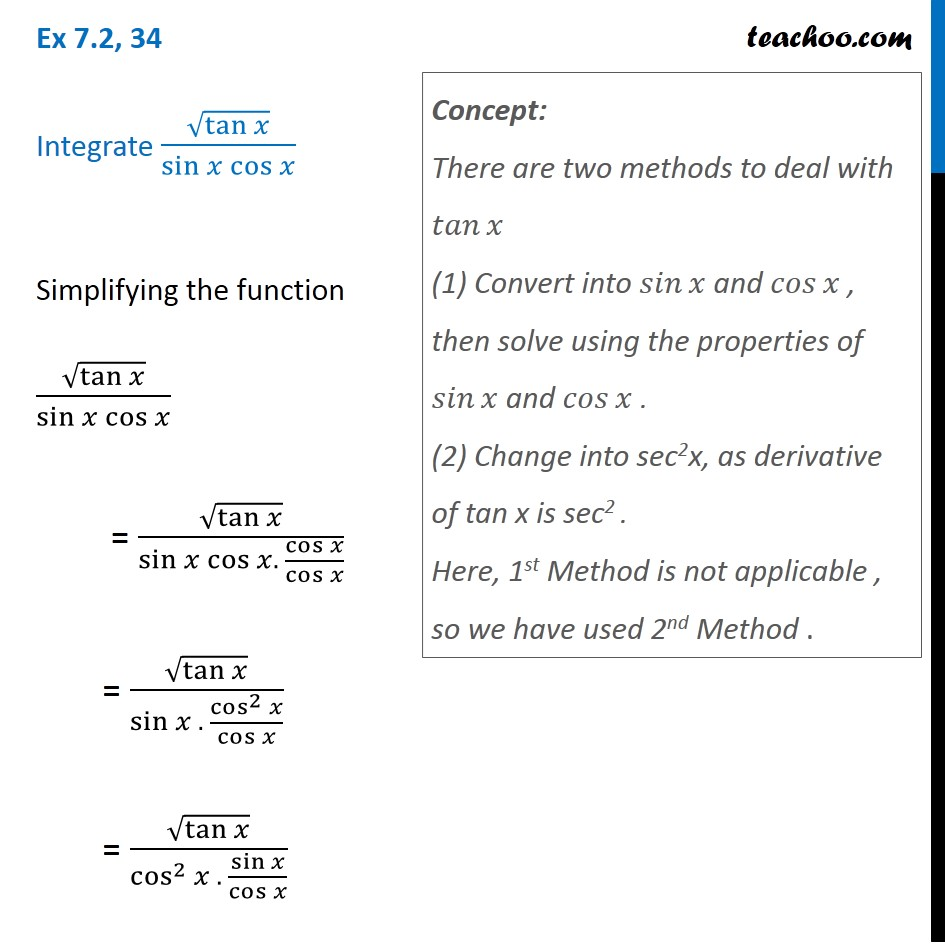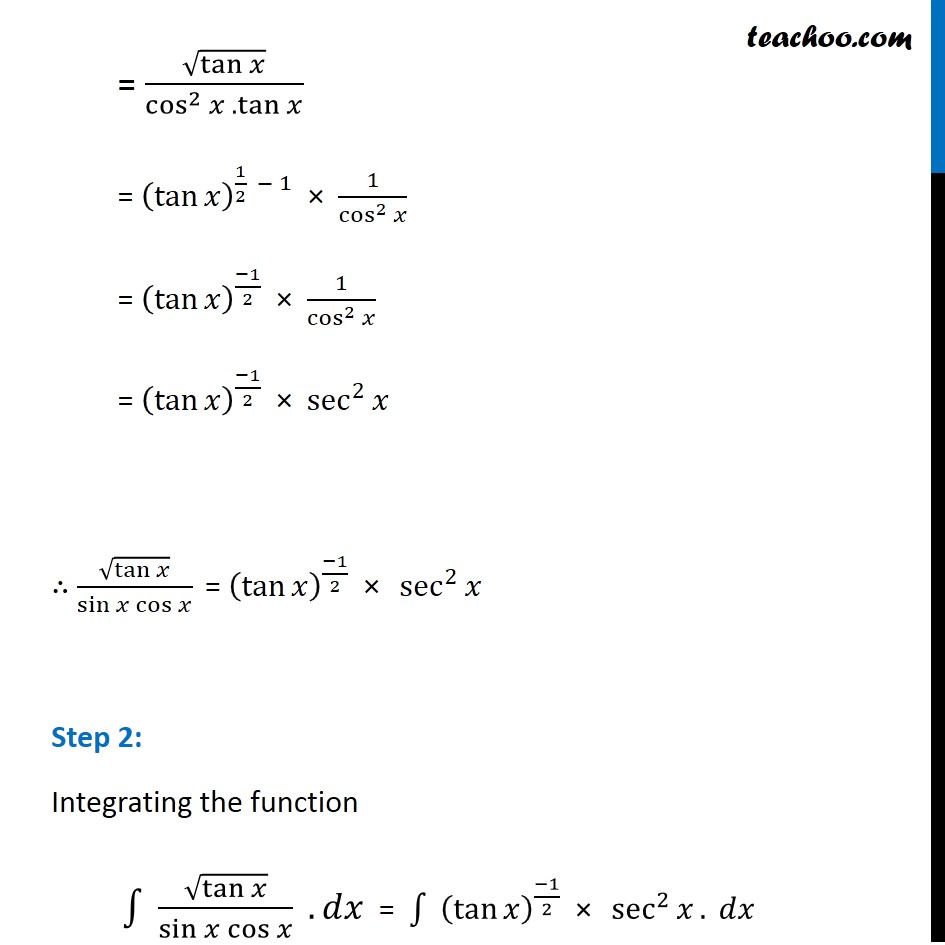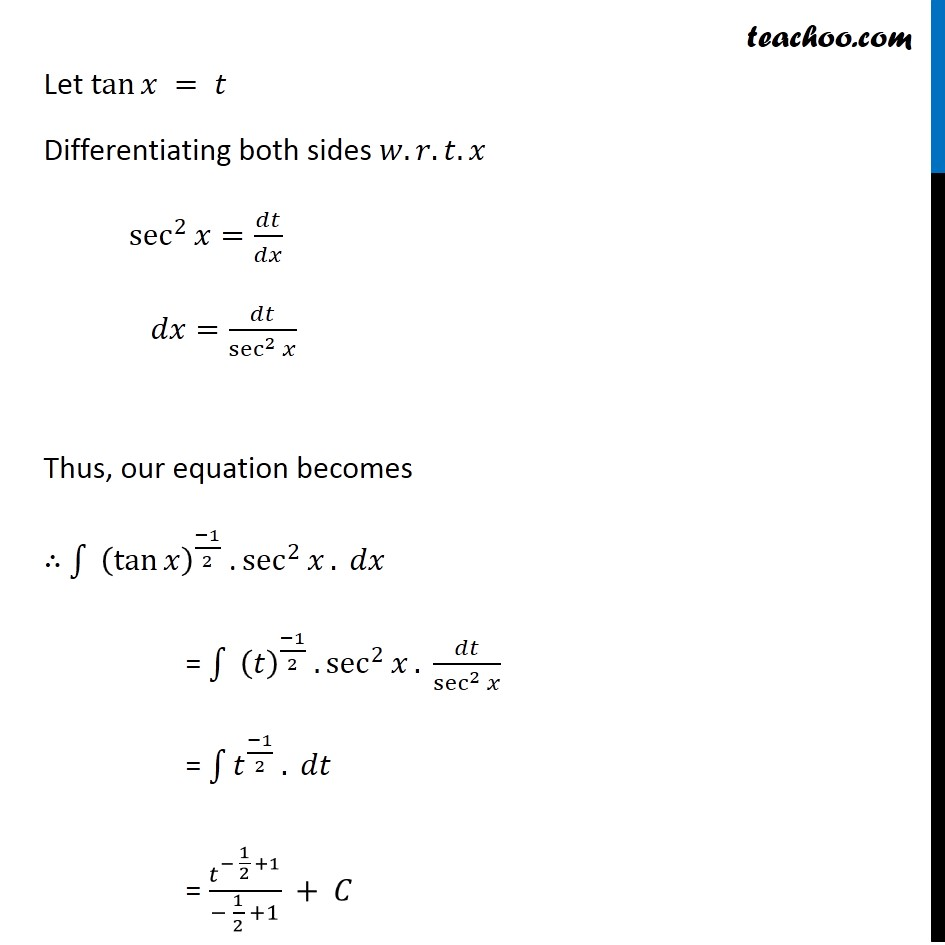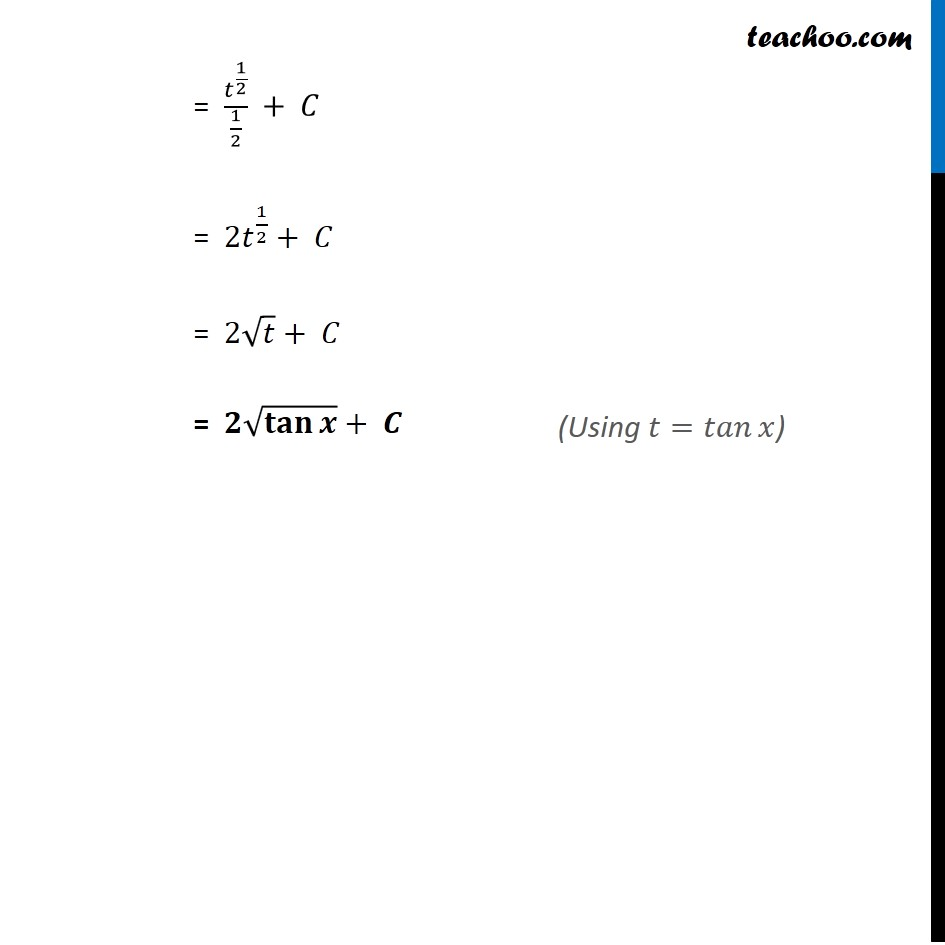Ex 7.2

Chapter 7 Class 12 Integrals
Serial order wiseLearn in your speed, with individual attention - Teachoo Maths 1-on-1 Class

### Transcript

Ex 7.2, 34 Integrate √(tan⁡𝑥 )/sin⁡〖𝑥 cos⁡𝑥 〗 Simplifying the function √(tan⁡𝑥 )/sin⁡〖𝑥 cos⁡𝑥 〗 = √(tan⁡𝑥 )/(sin⁡〖𝑥 cos⁡𝑥 〗. cos⁡𝑥/cos⁡𝑥 ) = √(tan⁡𝑥 )/(sin⁡𝑥 . cos^2⁡𝑥/cos⁡𝑥 ) = √(tan⁡𝑥 )/(cos^2⁡𝑥 . (sin 𝑥)/cos⁡𝑥 ) Concept: There are two methods to deal with 𝑡𝑎𝑛⁡𝑥 (1) Convert into 𝑠𝑖𝑛⁡𝑥 and 𝑐𝑜𝑠⁡𝑥 , then solve using the properties of 𝑠𝑖𝑛⁡𝑥 and 𝑐𝑜𝑠⁡𝑥 . (2) Change into sec2x, as derivative of tan x is sec2 . Here, 1st Method is not applicable , so we have used 2nd Method . = √(tan⁡𝑥 )/(cos^2⁡𝑥 . tan⁡𝑥 ) = (tan⁡𝑥 )^(1/2 − 1) × 1/cos^2⁡𝑥 = (tan⁡𝑥 )^((−1)/2) × 1/cos^2⁡𝑥 = (tan⁡𝑥 )^((−1)/2) × sec^2⁡𝑥 ∴ √(tan⁡𝑥 )/sin⁡〖𝑥 cos⁡𝑥 〗 " = " (tan⁡𝑥 )^((−1)/2) " × " sec^2⁡𝑥 Step 2: Integrating the function ∫1▒〖 √(tan⁡𝑥 )/sin⁡〖𝑥 cos⁡𝑥 〗 〗 . 𝑑𝑥 = ∫1▒〖 (tan⁡𝑥 )^((−1)/2) " × " sec^2⁡𝑥 〗. 𝑑𝑥" " Let tan⁡𝑥 = 𝑡 Differentiating both sides 𝑤.𝑟.𝑡.𝑥 sec^2⁡𝑥=𝑑𝑡/𝑑𝑥 𝑑𝑥=𝑑𝑡/sec^2⁡𝑥 Thus, our equation becomes ∴ ∫1▒〖 (tan⁡𝑥 )^((−1)/2) " ." sec^2⁡𝑥 〗. 𝑑𝑥" " = ∫1▒〖 (𝑡)^((−1)/2) " " . sec^2⁡𝑥 〗. 𝑑𝑡/sec^2⁡𝑥 " " = ∫1▒〖𝑡^((−1)/2) . 𝑑𝑡〗 = 𝑡^(− 1/2 +1)/(− 1/2 +1) + 𝐶 = 𝑡^(1/2)/(1/2) + 𝐶 = 〖2𝑡〗^(1/2)+ 𝐶 = 2√𝑡+ 𝐶 = 𝟐√(𝐭𝐚𝐧⁡𝒙 )+ 𝑪 (Using 𝑡=𝑡𝑎𝑛⁡𝑥)